Next Article in Journal
3D Ear Normalization and Recognition Based on Local Surface Variation
Erratum published on 22 February 2017, see Appl. Sci. 2017, 7(2), 211.
Open AccessArticle

# A CMOS Multiplied Input Differential Difference Amplifier: A New Active Device and Its Applications

1
Department of Radio Electronics, Faculty of Electrical Engineering and Communication, Brno University of Technology, Technicka 3082/12, Brno, Czech Republic
2
Department of Telecommunications, Faculty of Electrical Engineering and Communication, Brno University of Technology, Technicka 3082/12, Brno, Czech Republic
3
Department of Microelectronics, Faculty of Electrical Engineering and Communication, Brno University of Technology, Technicka 3058/10, Brno, Czech Republic
*
Author to whom correspondence should be addressed.
Appl. Sci. 2017, 7(1), 106; https://doi.org/10.3390/app7010106
Received: 9 November 2016 / Revised: 9 January 2017 / Accepted: 16 January 2017 / Published: 21 January 2017

## Abstract

This paper presents a newly developed active device, referred to as a multiplied input differential difference amplifier (MIDDA), which allows operations of summation/subtraction and multiplication of input signals. It was designed and fabricated using I3T25 0.35 μm ON (ON Semiconductor, Phoenix, AZ, USA) Semiconductor technology. The achieved results, which describe the experimentally verified behaviour of the fabricated device, are introduced, as well as the simple applications of MIDDA with electronically controllable parameters, useful for analogue signal processing. Moreover, the paper discusses an interesting example of nonlinear application to a double-sideband amplitude modulator, based on the utilization of multiplication and summation of particular signals. The laboratory experimental results which are achieved through the use of a fabricated prototype (both in time and frequency domain), confirm the workability of the concept.

## 1. Introduction

Active elements play an important role in communication subsystems, measurement, biomedical applications, and many other research areas. Standard active devices [1,2], commonly used in the latest research works, utilize linear operations in inter-terminal defining relations. This means that transfers between input and output terminals are ideally determined by frequency independent constants (voltage-, current-, transconductance-, or transresistance-gain/attenuation), or/and linear mathematic operations (summation or subtraction). The values of these constants can be electronically controlled in many cases. However, communication systems also require nonlinear operations in order to obtain common signal-processing blocks, such as mixers, modulators, etc. Attempts at trying to design these devices, implementing the so-called modular concept of internal structure, i.e., built from basic subparts with standard linear inter-terminal relations (current differencing transconductance amplifier , current conveyor transconductance amplifier , voltage differencing current conveyor [2,4], for example), have been reported. Devices with at least one nonlinear inter-terminal relation and their application potential, have not been frequently studied (it appears that there are only two works in recent literature [5,6]). The device presented in  employs commercially available components (a voltage-mode multiplier and current feedback amplifier). However, the proposed multiplication-mode current conveyor (MMCC) reported in [5,6], has some important drawbacks: (a) the MMCC element does not provide immediate results for the operation of multiplication in the form of current-sourced signal (therefore, it is not suitable for simple and direct creation of electronically controllable inverting/noninverting amplifiers, current-/voltage-mode integrators, etc., with a minimal number of external passive elements); (b) summation/subtraction operations are not directly available in the frame of the designed MMCC device (therefore, employment of the device as part of the chain in multi-feedback systems is much more difficult in the case of filters and oscillators). Our contribution presents an active device for direct providing the operations of multiplication, voltage summing, and subtraction, in a so-called multiplied input differential difference amplifier (MIDDA). A MIDDA addresses the issues previously noted, relating to MMCC elements. Its features are fully utilized in the nonlinear application of a double-sideband amplitude modulator (AM-DSB), that is not directly available with the help of previously reported MMCC elements (additional active circuitry must be included in order to obtain the same function, and therefore the final application is much more complex).

## 2. A Multiplied Input Differential Difference Amplifier

This active device was developed because of the requirement for electronically controllable active blocks (integrators, amplifiers, etc.), as well as the requirement for devices which provide a nonlinear operation of multiplication that is useful for analogue systems, as previously mentioned. A MIDDA partially operates as a voltage differencing differential difference amplifier (VDDDA), reported in [7,8]. However, the multiplication of signals at the input section is a new feature which is not provided by VDDDA elements. Figure 1 explains the small-signal behaviour of a MIDDA.
Figure 1a includes the block structure of a MIDDA. This topology was preserved in case of implementation using CMOS technology. The first subpart of a MIDDA, depicted in Figure 1a, is indicated by a MLT (multiplier) with a current output terminal. Note that our design was inspired by the solution presented in . However, it was completely redesigned, and supplemented by an input linearization system and a boosting operational transconductance amplifier [1,2]; this is visible in the full transistor structure, shown in Figure 2. When studying our design in detail, it is possible to observe some important differences to , as follows: (a) a redesign of the multiplying core, providing better dynamics and using larger bias current, allowing a wider range of the input voltage signal, as well as the speed and frequency bandwidth; (b) input linearizing attenuators, designed to improve applications with linear operations; and (c) an additional operational transconductance amplifier output section, required for the boosting of the output current and overall transconductance. The output operational transconductance amplifier was designed with cascoded current mirrors exhibiting auxiliary biasing (classical cascoded mirrors are not applicable in extremely low-voltage processes because of limited voltage space).
A new solution, which utilizes a CMOS voltage differential difference buffer (VDDB), forming the second subpart of the MIDDA (also shown in Figure 1a), differs from the classical concept of a VDDDA . As becomes obvious when studying the internal structure of the VDDB depicted in Figure 2, the CMOS circuitry is completely different, and much more complex, than the simple structures presented in [7,8]). The key difference is that our solution is based on folded-cascode architecture, and includes both NMOS and PMOS differential pairs. The CMOS process C035 I3T25 (ON Semiconductor) 0.35 μm (3.3 V) was chosen for the design and fabrication of the MIDDA prototype because it was the most suitable technology for our study. Figure 1b shows an overview of the layout of the fabricated device. Unfortunately, a microphotograph is not available due to technologically-unavoidable plating, placed above the fabricated die in I3T technologies. The MIDDA element has four voltage input terminals (Y, X, n, p), one output terminal o, and one auxiliary terminal z. The operation of the device can be defined using the following simple inter-terminal relations: Iz = VX × VY × k, Vo = VzVn + Vp, where k (given by technological constants and dimensions of key transistors in internal structures) can be expressed using:
$k ≅ ( 2 R a R b ) 2 . 2 K P n 2 ( W L ) 1 , 2 , 3 , 4 K P p 2 ( W L ) 5 , 6 R L .5 K P n 4 I b i a s 1 ( W L ) 7 , 8$
Design constants are: KPn = 136 μA/V2, KPp = 29 μA/V2, Vthn = 0.6 V, and Vthp = −0.62 V, for NMOS and PMOS in this I3T CMOS technology. The multiplying constant k was obtained from the detailed analysis of the MLT subpart. The first term of (1) represents the contribution of auxiliary linearization and attenuation blocks (components including MX1,2 and MY1,2 with resistors Ra and Rb, as visible from Figure 2). The second term of (1) covers the effect of differential pairs of the multiplying core (transistors M1–4 and M5–6 and their aspect ratios), and the conversion of the output current of these transistors, to the differential output voltage of the RL resistors. The last term (right side of (1)) includes the contribution of boosting the output transconductance amplifier (aspect ratio of M7,8 and 4Ibias1, derived from Ibias1 through biasing current mirrors, see Figure 2). Constant 5 represents the effect of the current gain of internal mirrors on the boosting transconductance amplifier. Note that a hand calculation of k yields a value of about −2 mA/V2, whereas a simulation provides a value of about −1.8 mA/V2 (note that the multiplier is inverting in basic configuration). This mismatch is caused by several inaccuracies in the ideal calculation: (a) a non-equal bulk and source voltage VBS ≠ 0 V for some transistors (differential pairs where bulk terminals are connected to VDD or VSS), which impacts the Vth and transconductance of partial specific transistors; (b) the rounding of resistor values (exact value supposes multiplication of 0.975 kΩ/square); (c) a process and temperature dependence of KP(n,p), as well as partial transconductances of CMOS transistors in proposed topology. The real experimental value of the k parameter equal to −1.3 mA/V2, falls into the range of the predicted values from Monte Carlo and corner analyses. The CMOS structure of the MIDDA element, including W/L aspect ratios of all the transistors, values of passive elements, and biasing sources, are shown in Figure 2. Note that not all ESD precautions and bulk connections (NMOS to VSS, PMOS to VDD) are included in Figure 2, because of simplicity.
The linear ranges of DC transfer characteristics of the real MLT section are limited up to ±500 mV for inputs X and Y, and up to ±350 μA for the auxiliary terminal z. The examples of measured DC transfer characteristics between input voltage (VY) and output current (IZ), are shown in Figure 3a; VX serves as the DC constant, driving overall transconductance (in accordance to gm ≅ 1.3 × 10−3 VX). The gain bandwidth (GBW) of the real prototype overcomes 48 MHz for the MLT subpart of the MIDDA (Figure 3b). The sweep of voltage VX between ±0.05 and ±0.5 V, causes a change in gm between ±60 μS and ±660 μS (both polarities of gm are available by the DC control voltage, in comparison to a standard operational transconductance amplifier ), as illustrated by a graph shown in Figure 4. The DC input and output linear range of VDDB reaches approximately ±700 mV (range is valid for inputs z, n, p, and output o), as shown in Figure 5a. The bandwidth (3 dB) of the VDDB part is 45.1 MHz or more, based on the particular configuration (see Figure 5b). Full, detailed information relating to the overall MIDDA performance is summarized in Table 1, including the results of the real experiments. It always covers the whole range of tunability for the controllable parameters. Even in the case when characteristics are asymmetrical in positive and negative corners, i.e., limits of linearity in positive and negative polarity are not symmetrical. Note that the ranges mentioned above are valid when symmetrical operation is required, i.e., absolute values of parameters are the same in both polarities in the case of the ranges mentioned above, which are the most useful in practice.
The examples of simple linear and nonlinear operations of the MIDDA are shown in Figure 6. Figure 6a presents the typical DC response of the MLT subpart, producing an output current Iz as a nonlinear parabolic function of the input voltages VX = VY = Vinp. This behaviour can be confirmed in the time domain (Figure 6b), where sine wave excitation creates output current with single polarity (negative due to negative k) and double frequency. Figure 6c,d provide an overview of the linear operation in time domain. The first figure represents the summation Vo = Vp + Vz (Figure 6c), and the second figure provides the results of the operation of subtraction Vo = VpVn (Figure 6d). In both cases, square-wave excitations were provided in order to also present the stability of the device. All of these results confirm VDDB functionality, as well as transient responses for sine wave excitation (Figure 6e). All accompanying details are included in Figure 6.
The proposed MIDDA is beneficial for utilization in linear, and especially nonlinear, applications. When a signal is connected to the Y terminal and a DC voltage is provided to the X terminal, this serves for a tuning of the overall transconductance gm (k × VX = gm), for example, the MIDDA usually operates in the linear applications. Nonlinear applications require a multiplier—both inputs (Y and X) are used for signal operations, as in the case of the AM modulator, for example.

## 3. Application Examples

The main intention of this paper is to introduce a MIDDA as a new active device, suitable for basic electronically controllable applications (amplifiers, integrators, and other building blocks required in analogue signal processing and circuit synthesis), and also as a building block for providing nonlinear operations. The following text focuses on the simple examples of MIDDA-based applications (controllable voltage amplifiers, controllable lossless integrators, controllable first-order high-pass filters, and double-sideband amplitude modulators). It should be highlighted that the presented building blocks, based on linear signal processing operations (amplifiers and integrators), possess features of electronic controllability in both polarities (they can be simply used in both the noninverting and inverting variant, by a simple change in the polarity of the DC control voltage).

#### 3.1. Simple Linear Applications (Linear Operation)

A simple circuit, representing a voltage-controlled voltage amplifier, is shown in Figure 7a. This amplifier is formed by using the MLT subpart of the MIDDA. This subpart creates a linear operation (VX input voltage serves for DC control of transconductance gm = k × VX, in Figure 7a labeled as Vgm). The second input Y serves as a signal input. Then, the input voltage Vinp transforms through the gm, to the output current from terminal z of the MLT subpart, and the external resistive load (R) provides a conversion of the current, back to voltage. The VDDB subpart serves as a simple voltage follower. The most important benefit of this application is its simple and immediate ability to change the polarity and value of the voltage gain by DC control voltage, very high input impedance, low output impedance, and a single grounded external passive component. The ideal value for the voltage gain of this amplifier can be determined as:
$K V _ a m p l = ± g m R = ± k V g m R ≅ ± 1.3 ⋅ 10 − 3 V g m R ,$
where gain range, dynamics, and frequency features, depend on an appropriate selection of value of external grounded resistance (R). Another possible application of a MIDDA is in a voltage-controlled lossless voltage integrator (Figure 7b). It is obtained by a simple change of the resistor (R), to a capacitor (C), as is obvious when comparing Figure 7a,b. This integrator is described in the following equation:
$K V _ int ( s ) = ± g m s C .$
This integrator has benefits that are similar to those of the voltage-controlled voltage amplifier discussed above. The benefits of both circuits in Figure 7 are not available in standard opamp-based solutions.
The last of the presented applications using linear signal processing is a first-order high-pass filter (Figure 8), directly allowing electronic tuning of the cut-off (pole) frequency. This application was obtained from previous topology, through the addition of simple feedback from the output, to terminal Y, and also by full utilization of the VDDB subpart. The symbolical transfer function has the following form:
$K V _ H P ( s ) = ± s s + g m s C$
Note that the filter can be configured as one which is either noninverting or inverting, when inputs (n, p) are interchanged, however, in case of the controllable voltage amplifier and voltage-controlled lossless integrator, polarity is controlled directly by the control voltage Vgm (see Figure 7).
The basic electronically controllable building blocks discussed above (for example integrators), are simple because of the direct implementation of the current output of the MLT subpart in the frame of the MIDDA, which allows it to omit at least one additional passive element. Moreover, it provides a low-impedance voltage output in these applications (voltage-controlled integrators, voltage-controlled amplifiers), which is an important advantage in comparison to [5,6].

#### 3.2. Simple Nonlinear Application (Nonlinear Operation)

The double-sideband amplitude modulator (AM-DSB) has been obtained by utilization of both the multiplicative and summing operations of the MIDDA, as illustrated in Figure 9. The presented solution also contains one resistor (R = 1 kΩ and voltage buffer (for impedance separation of spectrum analyser input having 50 Ω impedance)). Both of these supplementary components are present in the form of simple external parts. The modulating sine wave vm(t) has an amplitude of Vm = 200 mV, with a frequency of fm = 500 kHz connected to input Y, as an example. The carrier signal vc(t), with an amplitude of Vc = 250 mV, and a frequency of fc = 5 MHz, feeds into the input terminal X and auxiliary terminal p, simultaneously. A description of the operation will now be given (also shown in Figure 9b). The modulating wave signal vm(t) is multiplied with the carrier wave vc(t), and their product is available at the z terminal of the MLT subpart, in the form of voltage (after conversion of output current from the z terminal, to voltage, through the grounded resistor R). Following this, the VDDB subpart creates a summation of the carrier wave vc(t) with the product from the MLT subpart. The output voltage of the modulator is available in the simple form:
$v o ( t ) = v c ( t ) + v c ( t ) ⋅ v m ( t ) ⋅ k ⋅ R ,$
This formula can be modified to:
$v o ( t ) = V c [ 1 + k ⋅ R ⋅ v m ( t ) ] cos ( ω c t ) ,$
where vm(t) is the general (waveform shape) modulating signal and vc(t) = Vccos(ωct) represents the carrier wave. For the sine wave, we can rewrite (6) to:
$v o ( t ) = V c [ 1 + k ⋅ R ⋅ V m cos ( ω m t ) ] cos ( ω c t ) .$
The presence of the product k × R (R especially, k is technological constant) in the equations noted above can be used for variation of the amplitude ratio between modulation and the carrier wave. A similar effect can be observed when the simple attenuator between the input of carrier wave and p terminal is used. The features of this particular application of a MIDDA were verified experimentally. The results are shown in Figure 10. The modulation depth m obtained for the selected value of Vm and Vc (Figure 10a) reaches 25% (m = (Vo(max)Vo(min))/(Vo(max) + Vo(min)) × 100). A measured output spectrum is shown in Figure 10b. It can be seen that suppression of the closest spurious higher harmonics is above 47 dB. Overall power consumption of the MIDDA-based modulator is only 17 mW. Note that if the MIDDA is designed using more up-to-date technology (CMOS technology smaller than 0.35 µm), its power consumption is lower, however, its dynamic range available for signal processing is also significantly decreased. Therefore, the selection of a particular technology represents a trade-off that has to be accepted.

## 4. Discussion

Several advanced active devices with a various number of terminals and various principles of controllability have been developed in recent years [10,11,12,13,14,15,16,17,18,19,20,21,22,23]. Recently proposed active devices are compared in Table 2. However, only one of these exhibits the ability to perform multiplication between two input voltages, so acts as our MIDDA concept. MMCC device [5,6] has this operation implemented to voltage transfer between Y and X terminals of current conveyor of second generation (product of voltages from Y1 and Y2 terminals is available at X terminal). It may serve for the electronic control of voltage gain (A) between the Y and X terminals. However, as we stated in the introductory part of this paper, the MMCC does not simultaneously provide the results of multiplication in the form of current, and the summation/subtraction operations. Therefore, these limitations further complicate the implementation of the device in building blocks and applications discussed in this paper (for example in the case of the AM-DSB modulator).

## 5. Conclusions

The MIDDA device presented in this paper offers the following advantageous features, simultaneously: (a) the possibility to construct lossless, non/inverting voltage- or current-mode integrators with electronically controllable parameters, when only one external and grounded capacitor is added (useful for application in linear active filters, oscillators, and other subsystems)—to the best of authors’ knowledge, none of the commercially available multipliers have an accessible output response in the form of current; (b) the nonlinear operation of multiplication is available (for purposes of modulation, demodulation, mixing, shaping, etc., as was proven by this paper); (c) the direct availability of summation/subtraction operations (useful for multi-feedback systems); (d) it excludes utilization of opamp-based differential-summing amplifiers, including floating feedback resistors in the VDDB subpart, see internal structure of AD633, HA2556 , for example, due to a different concept of the CMOS structure; (e) comparable or better features to commercially available solutions (GBW, voltage ranges, offsets), even under lower supply voltages (±1.65 V). The proposed MIDDA device can be easily applied in miniaturized IC solutions for amplitude modulation and demodulation purposes, in long-wave and medium-wave communication systems. In comparison to [5,6], the MIDDA can be easily used for AM-DSB modulation (Figure 9), whereas MMCC suffers from the unavailability of output operation of summation of product of modulation and carrier wave with carrier wave. To the best of the authors’ knowledge, this device is the very first fabricated prototype based on the differential difference principle in the output section, and allows multiplication at the input section simultaneously.
We suppose that the future will see the development of many other (more complex) electronically controlled applications of MIDDA, in the field of harmonic oscillators (the useful methods for their synthesis are discussed in  for example), generators, multi-feedback active filters, and active filters, with electronically reconfigurable transfer responses [20,23], immittance converters, etc.

## Acknowledgments

This work was supported by the Czech Scientific Foundation (project 14-24186P). Research described in this paper was financed by the Czech Ministry of Education in the frame of the National Sustainability Program under grant LO1401. For research, infrastructure of the SIX Center was used.

## Author Contributions

R.S., R.P. and V.K. provided IC design and layout of fabricated device; R.S., J.J. and J.P. conceived, designed and performed the experiments; R.S. and J.J. wrote the paper.

## Conflicts of Interest

The authors declare no conflict of interest. The founding sponsors had no role in the design of the study; in the collection, analyses, or interpretation of data; in the writing of the manuscript, and in the decision to publish the results.

## References

1. Biolek, D.; Senani, R.; Biolkova, V.; Kolka, Z. Active elements for analog signal processing: Classification, review, and new proposals. Radioengineering 2008, 17, 15–32. [Google Scholar]
2. Senani, R.; Bhaskar, D.R.; Singh, A.K. Current Conveyors: Variants, Applications and Hardware Implementations, 1st ed.; Springer: Berlin/Heidelberg, Germany, 2015. [Google Scholar]
3. Biolek, D.; Biolkova, V. Allpass filter employing one grounded capacitor and one active element. Electron. Lett. 2009, 45, 807–808. [Google Scholar] [CrossRef]
4. Sotner, R.; Jerabek, J.; Prokop, R.; Kledrowetz, V. Simple CMOS voltage differencing current conveyor-based electronically tuneable quadrature oscillator. Electron. Lett. 2016, 52, 1016–1018. [Google Scholar] [CrossRef]
5. Hwang, Y.S.; Liu, W.H.; Tu, S.H.; Chen, J.J. New building block: Multiplication-mode current conveyor. IET Circ. Dev. Syst. 2009, 3, 41–48. [Google Scholar] [CrossRef]
6. Venkateswaran, P.; Nandi, R.; Das, S. New integrators and differentiators using MMCC. Circ. Syst. 2012, 3, 288–294. [Google Scholar] [CrossRef]
7. Herencsar, N.; Sotner, R.; Metin, B.; Koton, J.; Vrba, K. New ‘Voltage Differencing’ Device for Analog Signal Processing. In Proceedings of the 8th International Conference on Electrical and Electronics Engineering (ELECO), Bursa, Turkey, 28–30 November 2013; pp. 17–20.
8. Koton, J.; Herencsar, N.; Vrba, K.; Metin, B. Voltage-mode multifunction filter with mutually independent Q and w0 control feature using VDDDAs. Analog Integr. Circ. Signal Process. 2014, 81, 53–60. [Google Scholar] [CrossRef]
9. Jasielsky, J.; Kuta, S.; Machowski, W.; Kolodziejski, W. Four-quadrant CMOS transconductance multiplier operating at low voltage and high-speed. In Proceedings of the 17th International Conference on Mixed Design of Integrated Circuits and Systems (MIXDES), Wroclav, Poland, 14–26 June 2010; pp. 265–268.
10. Biolek, D. CDTA-building block for current-mode analog signal processing. In Proceedings of the European Conference on Circuit Theory and Design (ECCTD), Krakow, Poland, 1–4 September 2003; pp. 397–400.
11. Rai, S.-K.; Gupta, M. Current differencing transconductance amplifier (CDTA) with enhanced performance and its application. Analog Integr. Circ. Signal Process. 2016, 86, 307–319. [Google Scholar] [CrossRef]
12. Jaikla, W.; Siripruchyanun, M.; Bajer, J.; Biolek, D. A Simple Current-Mode Quadrature Oscillator Using Single CDTA. Radioengineering 2008, 17, 33–40. [Google Scholar]
13. Siripruchyanun, M.; Jaikla, W. CMOS current-controlled current differencing transconductance amplifier and applications to analog signal processing. AEU Int. J. Electron. Commun. 2008, 62, 277–287. [Google Scholar] [CrossRef]
14. Li, Y. Current-mode sixth-order elliptic band-pass filter using MCDTAs. Radioengineering 2011, 20, 645–649. [Google Scholar]
15. Prokop, R.; Musil, V. Modular approach to design of modern circuit blocks for current signal processing and new device CCTA. In Proceedings of the 7th International Conference on Signal and Image Processing (IASTED), Anaheim, CA, USA, 15–17 August 2005; pp. 494–499.
16. Prokop, R.; Musil, V. New modular current devices for true current mode signal processing. Electronics 2007, 16, 36–42. [Google Scholar]
17. Siripruchyanun, M.; Jaikla, W. Current controlled current conveyor transconductance amplifier (CCCCTA): A building block for analog signal processing. Electron. Eng. 2008, 90, 443–453. [Google Scholar] [CrossRef]
18. Herencsar, N.; Koton, J.; Vrba, K. Realisation of current mode KHN equivalent biquad using current follower amplifiers (CFTAs). IEICE Trans. Fund. Electron. Commun. Comput. Sci. 2010, E93-A, 1816–1819. [Google Scholar] [CrossRef]
19. Singh, B.; Singh, A.K.; Senani, R. New Universal Current-mode Biquad Using Only Three ZC-CFTAs. Radioengineering 2012, 21, 273–280. [Google Scholar]
20. Sotner, R.; Herencsar, N.; Jerabek, J.; Prokop, R.; Kartci, A.; Dostal, T.; Vrba, K. Z-Copy Controlled-Gain Voltage Differencing Current Conveyor: Advanced Possibilities in Direct Electronic Control of First-Order Filter. Elektron. Elektrotech. 2014, 20, 77–83. [Google Scholar] [CrossRef]
21. Sotner, R.; Jerabek, J.; Herencsar, N. Voltage Differencing Buffered/ Inverted Amplifiers and Their Applications for Signal Generation. Radioengineering 2013, 22, 490–504. [Google Scholar]
22. Sotner, R.; Jerabek, J.; Herencsar, N.; Zak, T.; Jaikla, W.; Vrba, K. Modified Current Differencing Unit and its Application for Electronically Reconfigurable Simple First-order Transfer Function. Adv. Electron. Comput. Eng. 2015, 15, 3–10. [Google Scholar] [CrossRef]
23. Sotner, R.; Jerabek, J.; Herencsar, N.; Prokop, R.; Lahiri, A.; Dostal, T.; Vrba, K. First-order transfer sections with reconnection-less electronically reconfigurable high-pass, all-pass and direct transfer character. J. Electron. Eng. 2016, 67, 12–20. [Google Scholar] [CrossRef]
24. Wang, H.-Y.; Tran, H.-D.; Nguyen, Q.-M.; Yin, L.-T.; Liu, C.-Y. Derivation of Oscillators from Biquadratic Band Pass Filters Using Circuit Transformations. Appl. Sci. 2014, 4, 482–492. [Google Scholar] [CrossRef]
Figure 1. Multiplied input differential difference amplifier (MIDDA) behavioural principles and subparts: (a) Symbol and internal sub-sections, ideal transfers; (b) Overview of the layout of cells (Cadence) of the fabricated prototype (0.31 mm2).
Figure 1. Multiplied input differential difference amplifier (MIDDA) behavioural principles and subparts: (a) Symbol and internal sub-sections, ideal transfers; (b) Overview of the layout of cells (Cadence) of the fabricated prototype (0.31 mm2).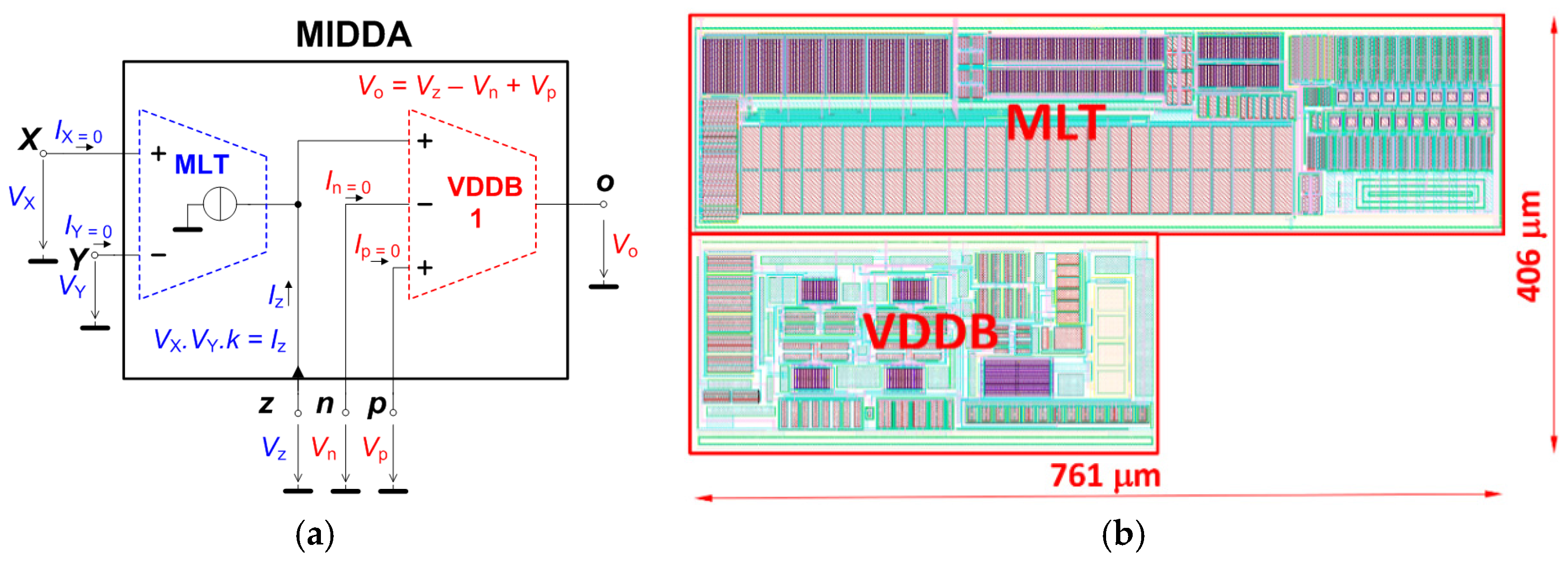Figure 2. MIDDA CMOS structure in ON Semiconductor C035 0.35 μm I3T25 technology.
Figure 2. MIDDA CMOS structure in ON Semiconductor C035 0.35 μm I3T25 technology.Figure 3. Measured characteristics of MLT (multiplier) with current output section in direction VYIZ (VX used for driving the transconductance by DC constant): (a) DC transfers; (b) AC magnitude responses.
Figure 3. Measured characteristics of MLT (multiplier) with current output section in direction VYIZ (VX used for driving the transconductance by DC constant): (a) DC transfers; (b) AC magnitude responses.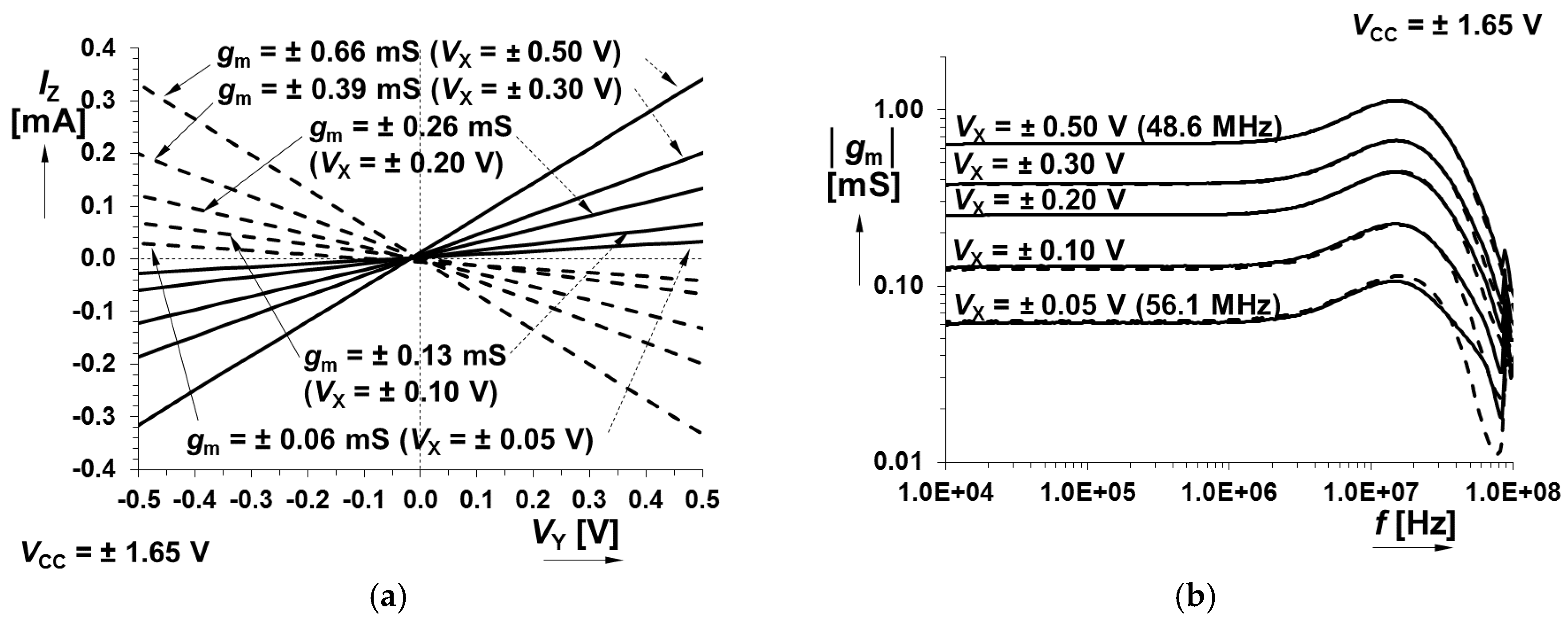Figure 4. Dependence of measured gm on VX (MLT stage of MIDDA).
Figure 4. Dependence of measured gm on VX (MLT stage of MIDDA).Figure 5. Measured characteristics of VDDB section: (a) DC transfers; (b) AC magnitude responses.
Figure 5. Measured characteristics of VDDB section: (a) DC transfers; (b) AC magnitude responses.Figure 6. A comparison of the simulated and measured examples of MIDDA nonlinear and linear basic operations: (a) MLT subpart DC transfer response of Iz at z terminal for VX = VY, i.e., squaring function; (b) MLT subpart transient output response Iz at z terminal; (c) VDDB subpart transient response of Vo at terminal o for summation Vo = Vp + Vz of two square waveforms; (d) VDDB subpart transient response of Vo at terminal o for subtraction Vo = VpVn of two square waveforms; (e) VDDB subpart transient response of Vo at terminal o for subtraction Vo = VpVn of two sine waveforms (measured only).
Figure 6. A comparison of the simulated and measured examples of MIDDA nonlinear and linear basic operations: (a) MLT subpart DC transfer response of Iz at z terminal for VX = VY, i.e., squaring function; (b) MLT subpart transient output response Iz at z terminal; (c) VDDB subpart transient response of Vo at terminal o for summation Vo = Vp + Vz of two square waveforms; (d) VDDB subpart transient response of Vo at terminal o for subtraction Vo = VpVn of two square waveforms; (e) VDDB subpart transient response of Vo at terminal o for subtraction Vo = VpVn of two sine waveforms (measured only).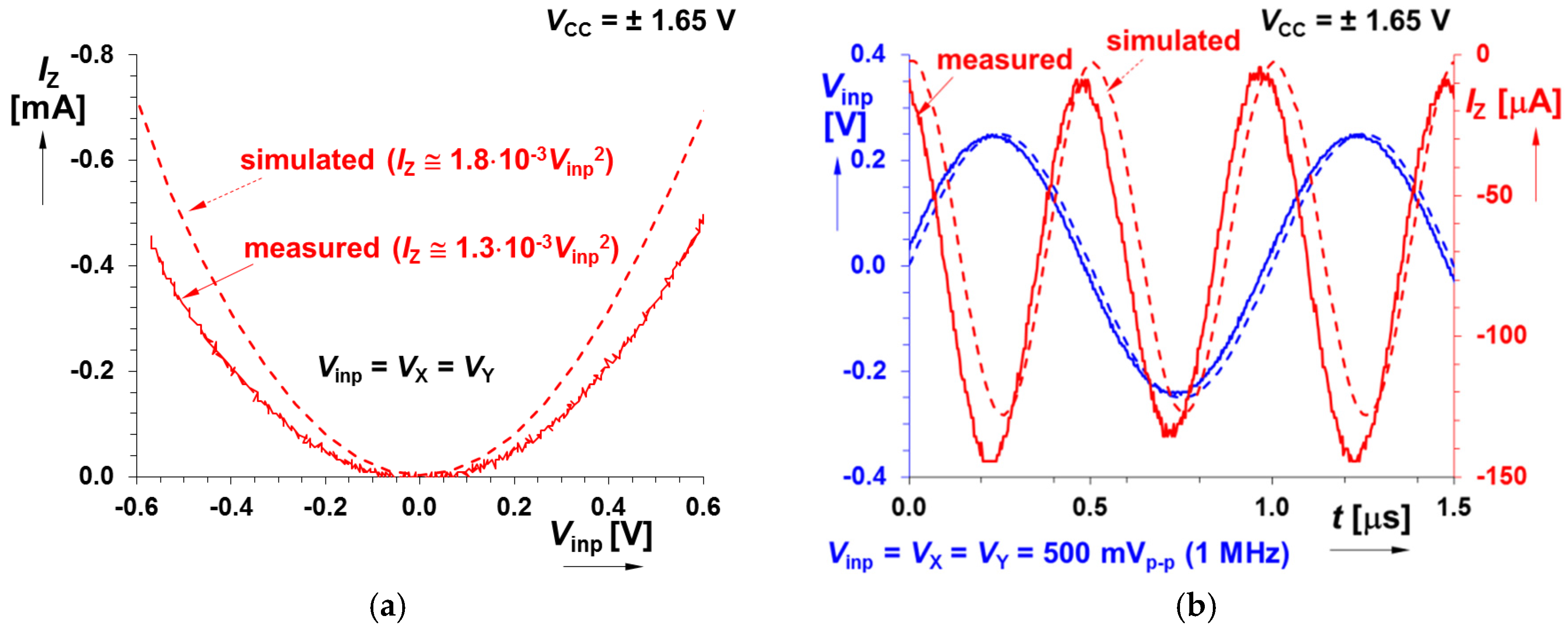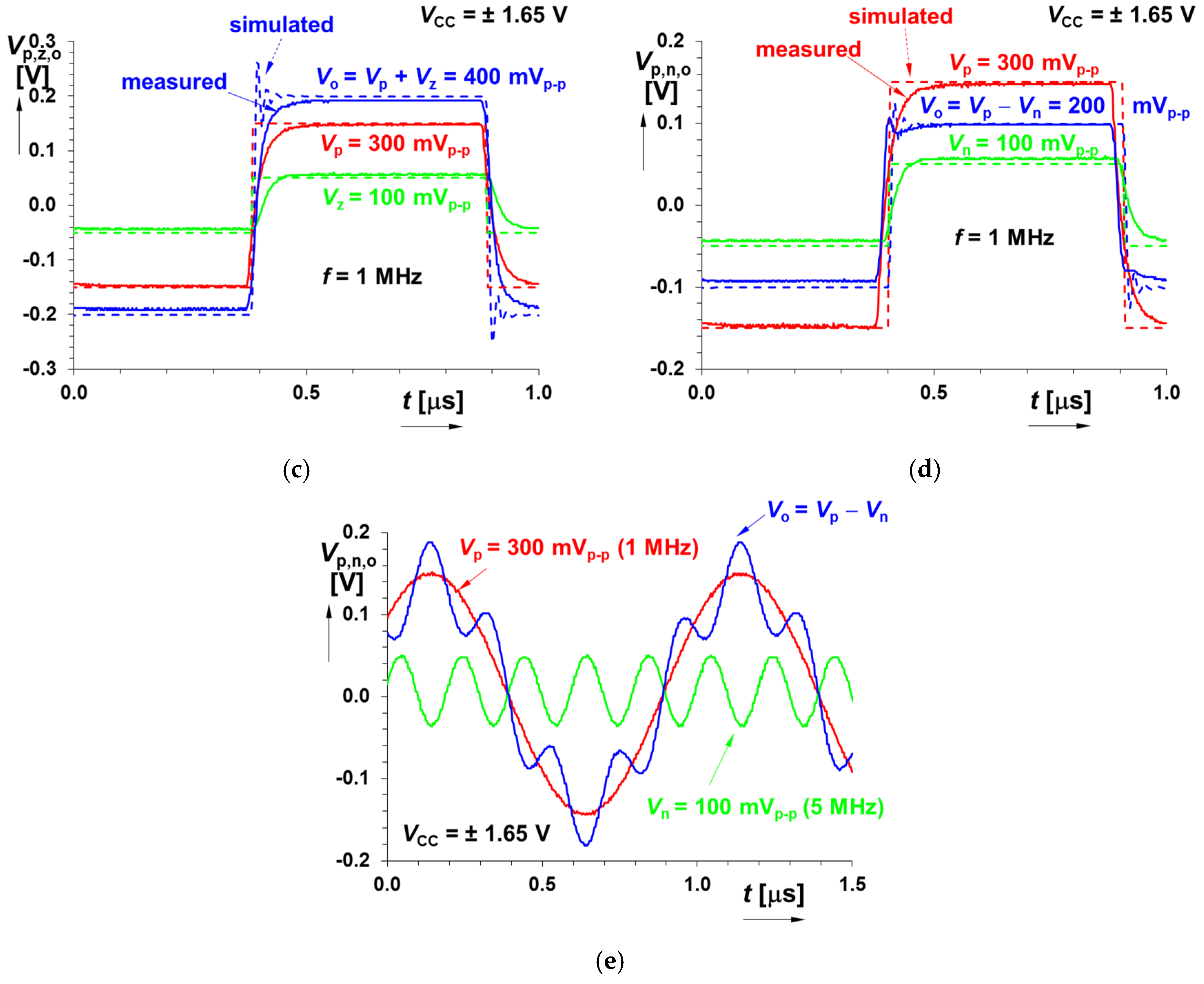Figure 7. Simple applications of a proposed MIDDA having voltage-controlled transfers in both polarities: (a) Voltage-controlled voltage amplifier; (b) Voltage-controlled lossless integrator.
Figure 7. Simple applications of a proposed MIDDA having voltage-controlled transfers in both polarities: (a) Voltage-controlled voltage amplifier; (b) Voltage-controlled lossless integrator.Figure 8. Simple application of a proposed MIDDA in a first-order high-pass filter with adjustable pole frequency.
Figure 8. Simple application of a proposed MIDDA in a first-order high-pass filter with adjustable pole frequency.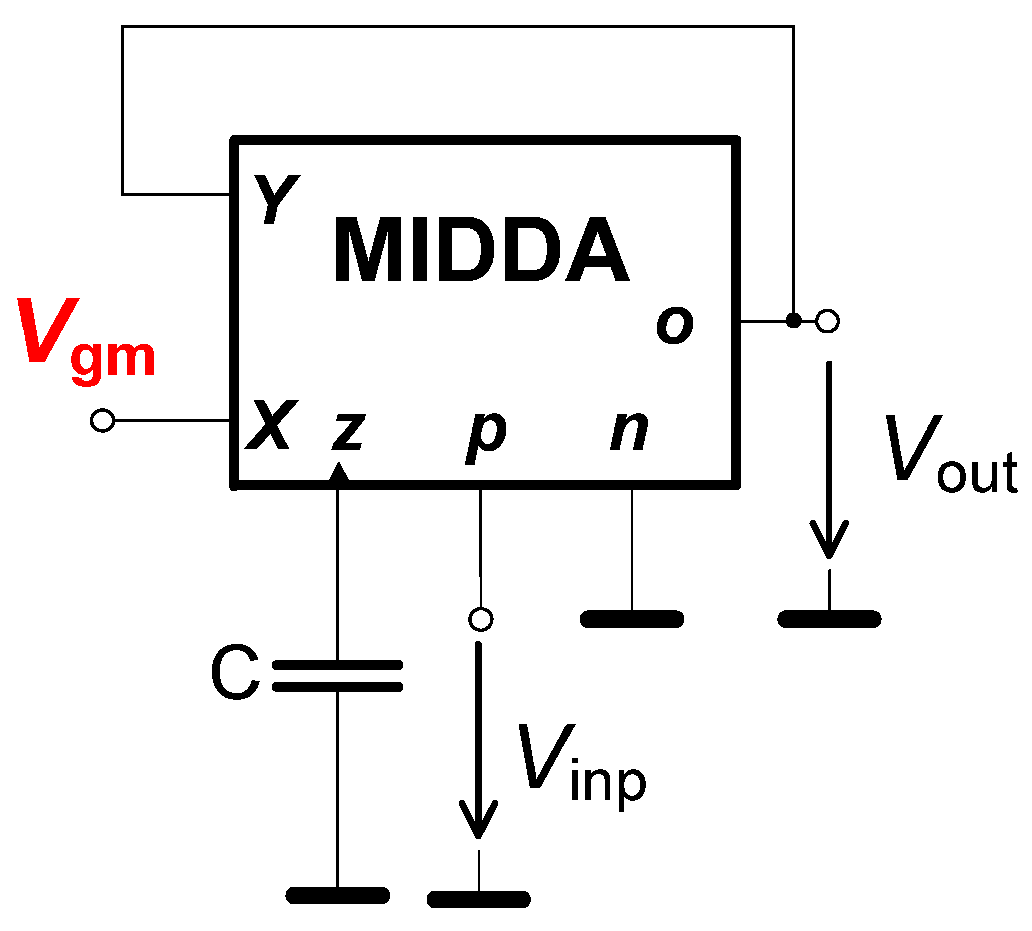Figure 9. Simple nonlinear application of a proposed MIDDA—AM-DSB modulator: (a) circuit implementation; (b) explanation of operation of modulation.
Figure 9. Simple nonlinear application of a proposed MIDDA—AM-DSB modulator: (a) circuit implementation; (b) explanation of operation of modulation.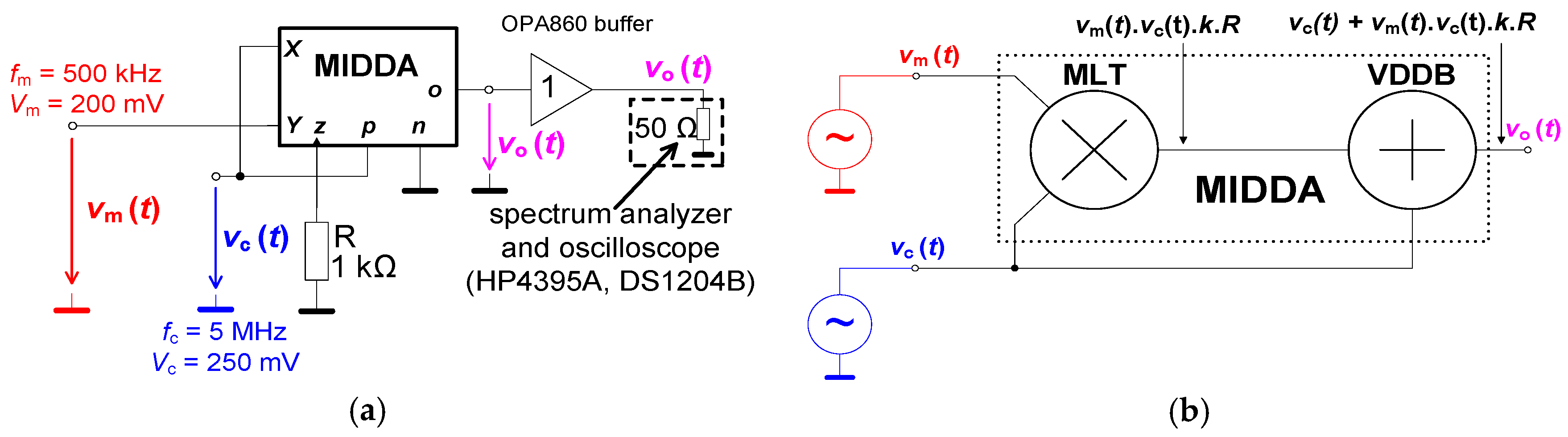Figure 10. Example of measured output response of the AM-DSB modulator with 5 MHz carrier frequency and modulation depth m = 25%: (a) time domain; (b) frequency domain (output spectrum).
Figure 10. Example of measured output response of the AM-DSB modulator with 5 MHz carrier frequency and modulation depth m = 25%: (a) time domain; (b) frequency domain (output spectrum).Table 1. Overall typical performance of MIDDA obtained from the measurements.
Table 1. Overall typical performance of MIDDA obtained from the measurements.
CMOS MLT Subpart
Parameter/Transfer from→to
Small-Signal AC Transfer (GBW > 42 MHz)
gm (Xz) (VY = 50→800 mV)45→974 μS
gm (Xz) (VY = −50→−800 mV)−80→−2210 μS
gm (Yz) (VX = 50→800 mV)60→1030 μS
gm (Yz) (VX = −50→−800 mV)−62→−1700 μS
input DC dynamical range
Xz (VY = ±50→±500 mV)−500→900 mV
Yz (VX = ±50→±500 mV)−600→900 mV
harmonic distortion
THDXz (1 kHz, VY = ±1000 mV)0.06%→1.08% (for VX = 200→1000 mVpk-pk)
THDYz (1 kHz, VX = ±1000 mV)0.08%→1.38% (for VY = 200→700 mVpk-pk)
input/output resistances
RX_DC (any value of VY)100 MΩ
RY_DC (any value of VX)100 MΩ
Rz_DC (VY = 50→800 mV)1 MΩ→176 kΩ
Rz_DC (VY = −50→−800 mV)3 MΩ→140 kΩ
Rz_DC (VX = 50→800 mV)66 kΩ→5.2 kΩ
Rz_DC (VX = −50→−800 mV)107 kΩ→2.4 kΩ
CMOS VDDB Subpart
small-signal AC transfer
KzO [−] (−3 dB)1.02 (55 MHz)
KnO [−] (−3 dB)1.02 (62 MHz)
KpO [−] (−3 dB)1.01 (45 MHz)
input dynamical range
zo−800→700 mV
no−700→700 mV
po−1600→1000 mV
distortion
THDzo0.04%→0.41% (for Vz = 100→1500 mVpk-pk)
THDno0.07%→0.33% (for Vn = 100→1500 mVpk-pk)
THDpo0.03%→0.11% (for Vp = 100→1500 mVpk-pk)
input/output resistances
Rz,n,p100 MΩ
RO0.54 Ω
Table 2. Comparison of recently reported electronically controllable advanced multi-terminal active devices (selected examples).
Table 2. Comparison of recently reported electronically controllable advanced multi-terminal active devices (selected examples).
WorkActive DeviceNo. of Terminals fNo. of Controllable Parameters (Type)MULTIPLICATIVE Inter-Terminal Relation AvailableDevice Fabricated as IC
[1,3,10,11,12]CDTA 151 (gm) aNoYes
CCCDTA 243 (Rp, Rn, gm) bNoNo
MCDTA 382 (gm1, gm2)NoNo
[15,16]CCTA 441 (gm)NoYes
CCCCTA 542 (RX, gm)NoNo
[18,19]CFTA, ZC-CFTA 64 (5)1 (gm)NoNo
[4,20]VDCC, ZC-CG-VDCC 76 (7)2 (RX, gm)
3 (RX, gm, B) c
NoYes
DO-VDBA 851 (gm)NoNo
FB-VDBA 961 (gm)
DO-CG-VDBVA 1062 (gm, A) d
MCDU 1154 (Rp, Rn, B1, B2)NoNo
ZC-CCCFDITA 1262 (Rf, gm)NoNo
[7,8]VDDDA 1361 (gm)NoNo
[5,6]MMCC 1441 (A) eYesNo
this workMIDDA61 (gm) eYesYes
1 Current Differencing Transconductance Amplifier (CDTA); 2 Current Controlled CDTA (CCCDTA); 3 Modified CDTA (MCDTA); 4 Current Conveyor Transconductance Amplifier (CCTA); 5 Current Controlled CCTA; 6 (Z-copy) Current Follower Transconductance Amplifier ((ZC)-CFTA); 7 (Z-copy Controlled-Gain) Voltage Differencing Current Conveyor (VDCC, (ZC-CG)-VDCC); 8 Dual Output Voltage Differencing Buffered Amplifier (DO-VDBA); 9 Fully Balanced VDBA (FB-VDBA); 10 Dual Output Controlled Gain Voltage Differencing Buffered Voltage Amplifier (DO-CG-VDBVA); 11 Modified Current Differencing Unit (MCDU); 12 Z-copy Current Controlled Current Followed Differential Input Transconductance Amplifier (ZC-CCCFDITA); 13 Voltage Differencing Differential Difference Amplifier (VDDDA); 14 Multiplication Mode Current Conveyor (MMCC); a transconductance (gm); b electronically adjustable resistance of single current input terminal (RX, Rf) or resistances of differential input terminals (Rp, Rn); c electronically adjustable current gain (B); d electronically adjustable voltage gain (A); e available if one of the input voltages of the multiplier section is supposed as the DC constant (if necessary for linear inter-terminal operation); f terminals for DC control of parameters not included.